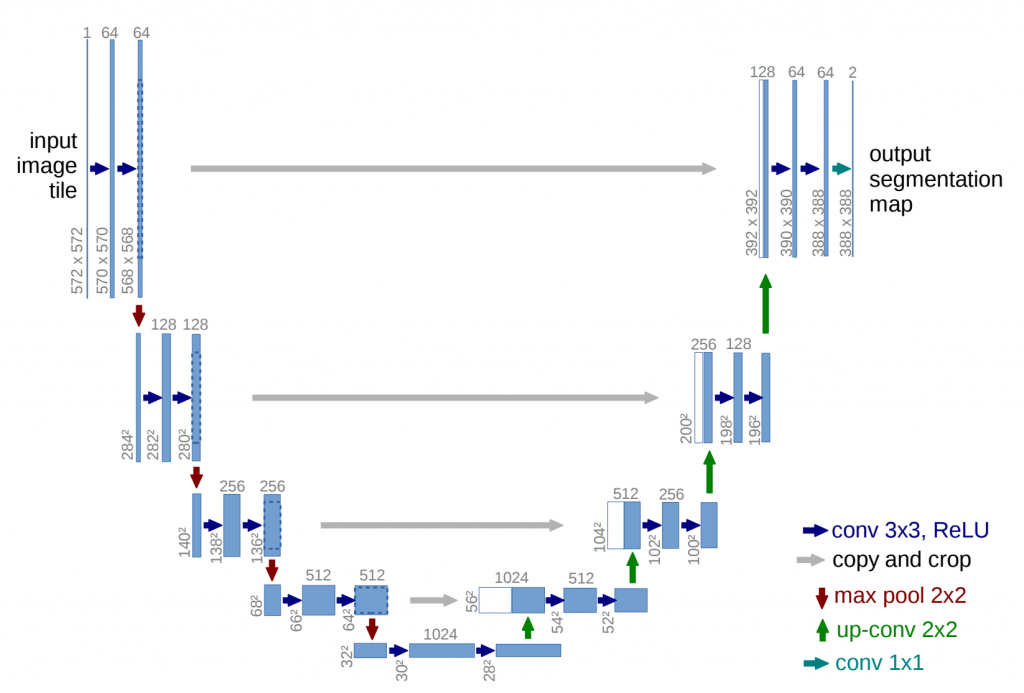#DAY 14
1
AI & Data

## 架構The network architecture is illustrated in Figure 1. It consists of a contracting path (left side) and an expansive path (right side).

The contracting path follows the typical architecture of a convolutional network. It consists of the repeated application of two 3x3 convolutions (unpadded convolutions), each followed by a rectified linear unit (ReLU) and a 2x2 max pooling operation with stride 2 for downsampling. At each downsampling step we double the number of feature channels.

1. 3X3 架構的convolution *2
2. relu 激活函數
3. pooling * 1

Every step in the expansive path consists of an upsampling of the feature map followed by a 2x2 convolution ("up-convolution") that halves the number of feature channels, a concatenation with the correspondingly cropped feature map from the contracting path, and two 3x3 convolutions, each followed by a ReLU.

1. 通道數減半的向上convolution (取代pooling)
2. convolution 同左邊架構

The cropping is necessary due to the loss of border pixels in every convolution. At the final layer a 1x1 convolution is used to map each 64-component feature vector to the desired number of classes. In total the network has 23 convolutional layers. To allow a seamless tiling of the output segmentation map (see Figure 2), it is important to select the input tile size such that all 2x2 max-pooling operations are applied to a layer with an even x- and y-size.

1. 每次都會丟失邊界，所以要將影像再還原回去

 U-net

30天只學U-net30